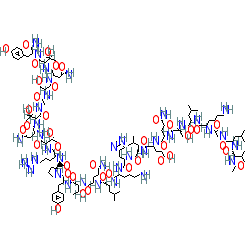#### GtoPdb Ligand ID: 689

Synonyms: AC 187 | AC-187 | acetyl-(Asn30,Tyr32)-calcitonin8-32 | salmon calcitonin (8-32) reduced2D StructureSMILES / InChI / InChIKeyCanonical SMILES NCCCCC(C(=O)NC(C(=O)NC(C(=O)NC(C(=O)NC(C(=O)N1CCCC1C(=O)NC(C(=O)NC(C(=O)NC(C(=O)NC(C(=O)NCC(=O)NC(C(=O)NC(C(=O)NC(C(=O)NC(C(=O)N)Cc1ccc(cc1)O)C(O)C)CC(=O)N)CO)C(O)C)CC(=O)N)C(O)C)CCCNC(=N)N)Cc1ccc(cc1)O)C(O)C)CCC(=O)N)CC(C)C)NC(=O)C(NC(=O)C(NC(=O)C(NC(=O)C(NC(=O)C(NC(=O)C(NC(=O)C(NC(=O)CNC(=O)C(NC(=O)C(C(C)C)NC(=O)C)CC(C)C)CCCCN)CC(C)C)CO)CCC(=O)N)CCC(=O)O)CC(C)C)Cc1nc[nH]c1 Isomeric SMILES NCCCC[C@@H](C(=O)N[C@H](C(=O)N[C@H](C(=O)N[C@H](C(=O)N[C@H](C(=O)N1CCC[C@H]1C(=O)N[C@H](C(=O)N[C@H](C(=O)N[C@H](C(=O)N[C@H](C(=O)NCC(=O)N[C@H](C(=O)N[C@H](C(=O)N[C@H](C(=O)N[C@H](C(=O)N)Cc1ccc(cc1)O)[C@H](O)C)CC(=O)N)CO)[C@H](O)C)CC(=O)N)[C@H](O)C)CCCNC(=N)N)Cc1ccc(cc1)O)[C@H](O)C)CCC(=O)N)CC(C)C)NC(=O)[C@@H](NC(=O)[C@@H](NC(=O)[C@@H](NC(=O)[C@@H](NC(=O)[C@@H](NC(=O)[C@@H](NC(=O)[C@@H](NC(=O)CNC(=O)[C@@H](NC(=O)[C@H](C(C)C)NC(=O)C)CC(C)C)CCCCN)CC(C)C)CO)CCC(=O)N)CCC(=O)O)CC(C)C)Cc1nc[nH]c1 InChI InChI=1S/C127H205N37O40/c1-59(2)44-81(156-122(200)99(63(9)10)142-68(15)171)105(183)139-54-96(178)143-74(22-16-18-40-128)106(184)151-84(47-62(7)8)114(192)159-90(57-166)119(197)148-77(34-37-92(130)174)108(186)146-79(36-39-98(180)181)109(187)153-83(46-61(5)6)113(191)154-85(50-71-53-137-58-141-71)115(193)145-75(23-17-19-41-129)107(185)152-82(45-60(3)4)112(190)147-78(35-38-93(131)175)111(189)162-103(67(14)170)125(203)158-88(49-70-28-32-73(173)33-29-70)126(204)164-43-21-25-91(164)120(198)149-76(24-20-42-138-127(135)136)110(188)161-102(66(13)169)124(202)157-87(52-95(133)177)117(195)160-100(64(11)167)121(199)140-55-97(179)144-89(56-165)118(196)155-86(51-94(132)176)116(194)163-101(65(12)168)123(201)150-80(104(134)182)48-69-26-30-72(172)31-27-69/h26-33,53,58-67,74-91,99-103,165-170,172-173H,16-25,34-52,54-57,128-129H2,1-15H3,(H2,130,174)(H2,131,175)(H2,132,176)(H2,133,177)(H2,134,182)(H,137,141)(H,139,183)(H,140,199)(H,142,171)(H,143,178)(H,144,179)(H,145,193)(H,146,186)(H,147,190)(H,148,197)(H,149,198)(H,150,201)(H,151,184)(H,152,185)(H,153,187)(H,154,191)(H,155,196)(H,156,200)(H,157,202)(H,158,203)(H,159,192)(H,160,195)(H,161,188)(H,162,189)(H,163,194)(H,180,181)(H4,135,136,138)/t64-,65-,66-,67-,74+,75+,76+,77+,78+,79+,80+,81+,82+,83+,84+,85+,86+,87+,88+,89+,90+,91+,99+,100+,101+,102+,103+/m1/s1 InChI Key ZLFXHYNEZYAYPG-AABHONRUSA-N